# 3.4 Graphs of polynomial functions  (Page 9/13)

 Page 9 / 13

$f\left(x\right)={x}^{5}-5{x}^{3}+4x$

For the following exercises, use the Intermediate Value Theorem to confirm that the given polynomial has at least one zero within the given interval.

$f\left(x\right)={x}^{3}-9x,\text{\hspace{0.17em}}$ between $\text{\hspace{0.17em}}x=-4\text{\hspace{0.17em}}$ and $\text{\hspace{0.17em}}x=-2.$

$f\left(x\right)={x}^{3}-9x,\text{\hspace{0.17em}}$ between $\text{\hspace{0.17em}}x=2\text{\hspace{0.17em}}$ and $\text{\hspace{0.17em}}x=4.$

$f\left(2\right)=–10\text{\hspace{0.17em}}$ and $\text{\hspace{0.17em}}f\left(4\right)=28.$ Sign change confirms.

$f\left(x\right)={x}^{5}-2x,\text{\hspace{0.17em}}$ between $\text{\hspace{0.17em}}x=1\text{\hspace{0.17em}}$ and $\text{\hspace{0.17em}}x=2.$

$f\left(x\right)=-{x}^{4}+4,\text{\hspace{0.17em}}$ between $\text{\hspace{0.17em}}x=1\text{\hspace{0.17em}}$ and $\text{\hspace{0.17em}}x=3$ .

$f\left(1\right)=3\text{\hspace{0.17em}}$ and $\text{\hspace{0.17em}}f\left(3\right)=–77.\text{\hspace{0.17em}}$ Sign change confirms.

$f\left(x\right)=-2{x}^{3}-x,\text{\hspace{0.17em}}$ between $\text{\hspace{0.17em}}x=–1\text{\hspace{0.17em}}$ and $\text{\hspace{0.17em}}x=1.$

$f\left(x\right)={x}^{3}-100x+2,\text{\hspace{0.17em}}$ between $\text{\hspace{0.17em}}x=0.01\text{\hspace{0.17em}}$ and $\text{\hspace{0.17em}}x=0.1$

$f\left(0.01\right)=1.000001\text{\hspace{0.17em}}$ and $\text{\hspace{0.17em}}f\left(0.1\right)=–7.999.\text{\hspace{0.17em}}$ Sign change confirms.

For the following exercises, find the zeros and give the multiplicity of each.

$f\left(x\right)={\left(x+2\right)}^{3}{\left(x-3\right)}^{2}$

$f\left(x\right)={x}^{2}{\left(2x+3\right)}^{5}{\left(x-4\right)}^{2}$

0 with multiplicity 2, $\text{\hspace{0.17em}}-\frac{3}{2}\text{\hspace{0.17em}}$ with multiplicity 5, 4 with multiplicity 2

$f\left(x\right)={x}^{3}{\left(x-1\right)}^{3}\left(x+2\right)$

$f\left(x\right)={x}^{2}\left({x}^{2}+4x+4\right)$

0 with multiplicity 2, –2 with multiplicity 2

$f\left(x\right)={\left(2x+1\right)}^{3}\left(9{x}^{2}-6x+1\right)$

$f\left(x\right)={\left(3x+2\right)}^{5}\left({x}^{2}-10x+25\right)$

$-\frac{2}{3}\text{\hspace{0.17em}}\text{with}\text{\hspace{0.17em}}\text{multiplicity}\text{\hspace{0.17em}}5\text{,}\text{\hspace{0.17em}}5\text{\hspace{0.17em}}\text{with}\text{\hspace{0.17em}}\text{multiplicity}\text{\hspace{0.17em}}\text{2}$

$f\left(x\right)=x\left(4{x}^{2}-12x+9\right)\left({x}^{2}+8x+16\right)$

$f\left(x\right)={x}^{6}-{x}^{5}-2{x}^{4}$

$\text{0}\text{\hspace{0.17em}}\text{with}\text{\hspace{0.17em}}\text{multiplicity}\text{\hspace{0.17em}}4\text{,}\text{\hspace{0.17em}}2\text{\hspace{0.17em}}\text{with}\text{\hspace{0.17em}}\text{multiplicity}\text{\hspace{0.17em}}1\text{,}\text{\hspace{0.17em}}–\text{1}\text{\hspace{0.17em}}\text{with}\text{\hspace{0.17em}}\text{multiplicity}\text{\hspace{0.17em}}1$

$f\left(x\right)=3{x}^{4}+6{x}^{3}+3{x}^{2}$

$f\left(x\right)=4{x}^{5}-12{x}^{4}+9{x}^{3}$

$\frac{3}{2}\text{\hspace{0.17em}}$ with multiplicity 2, 0 with multiplicity 3

$f\left(x\right)=2{x}^{4}\left({x}^{3}-4{x}^{2}+4x\right)$

$f\left(x\right)=4{x}^{4}\left(9{x}^{4}-12{x}^{3}+4{x}^{2}\right)$

$\text{0}\text{\hspace{0.17em}}\text{with}\text{\hspace{0.17em}}\text{multiplicity}\text{\hspace{0.17em}}6\text{,}\text{\hspace{0.17em}}\frac{2}{3}\text{\hspace{0.17em}}\text{with}\text{\hspace{0.17em}}\text{multiplicity}\text{\hspace{0.17em}}2$

## Graphical

For the following exercises, graph the polynomial functions. Note $\text{\hspace{0.17em}}x\text{-}$ and $\text{\hspace{0.17em}}y\text{-}$ intercepts, multiplicity, and end behavior.

$f\left(x\right)={\left(x+3\right)}^{2}\left(x-2\right)$

$g\left(x\right)=\left(x+4\right){\left(x-1\right)}^{2}$

x -intercepts, $\left(1, 0\right)$ with multiplicity 2, $\left(–4, 0\right)$ with multiplicity 1, $y\text{-}$ intercept $\left(0, 4\right)$ . As $x\to -\infty$ , $f\left(x\right)\to -\infty$ , as $x\to \infty$ , $f\left(x\right)\to \infty$ .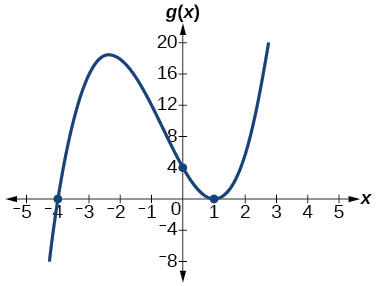$h\left(x\right)={\left(x-1\right)}^{3}{\left(x+3\right)}^{2}$

$k\left(x\right)={\left(x-3\right)}^{3}{\left(x-2\right)}^{2}$

x -intercepts $\left(3,0\right)$ with multiplicity 3, $\left(2,0\right)$ with multiplicity 2, $y\text{-}$ intercept $\left(0,–108\right)$ . As $x\to -\infty$ , $f\left(x\right)\to -\infty$ , as $x\to \infty$ , $f\left(x\right)\to \infty .$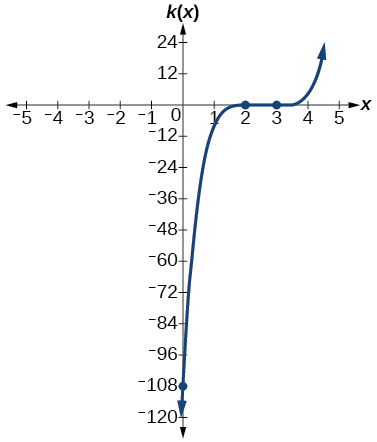$m\left(x\right)=-2x\left(x-1\right)\left(x+3\right)$

$n\left(x\right)=-3x\left(x+2\right)\left(x-4\right)$

x -intercepts $\left(0, 0\right),\phantom{\rule{0.2em}{0ex}}\left(–2, 0\right),\phantom{\rule{0.2em}{0ex}}\left(4, 0\right)$ with multiplicity 1, $y$ -intercept $\left(0, 0\right).$ As $x\to -\infty$ , $f\left(x\right)\to \infty$ , as $x\to \infty$ , $f\left(x\right)\to -\infty .$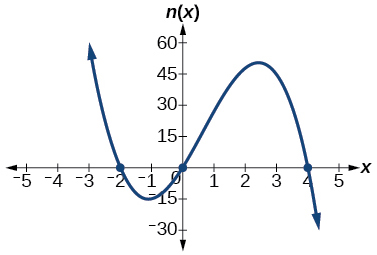For the following exercises, use the graphs to write the formula for a polynomial function of least degree.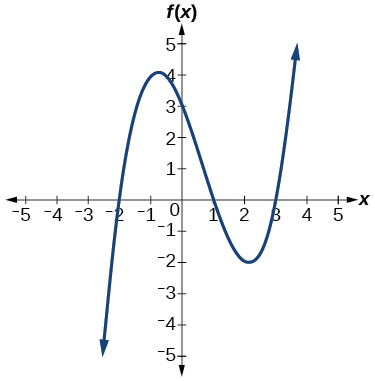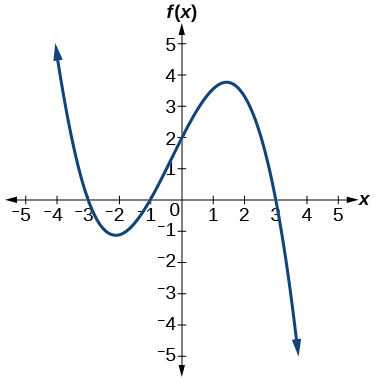$f\left(x\right)=-\frac{2}{9}\left(x-3\right)\left(x+1\right)\left(x+3\right)$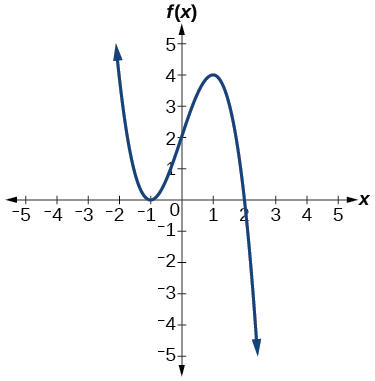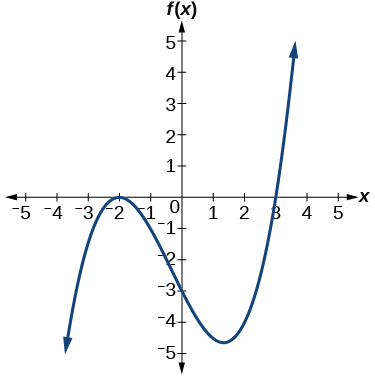$f\left(x\right)=\frac{1}{4}{\left(x+2\right)}^{2}\left(x-3\right)$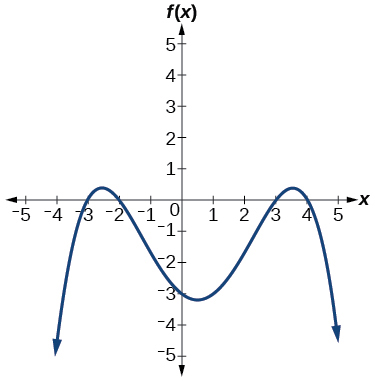For the following exercises, use the graph to identify zeros and multiplicity.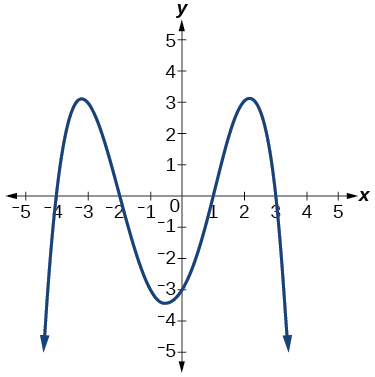–4, –2, 1, 3 with multiplicity 1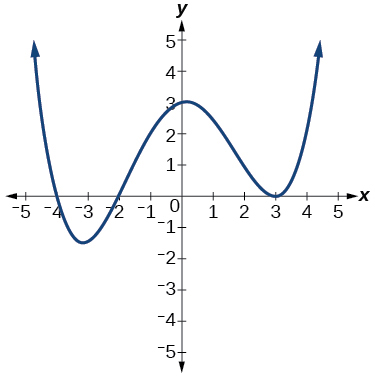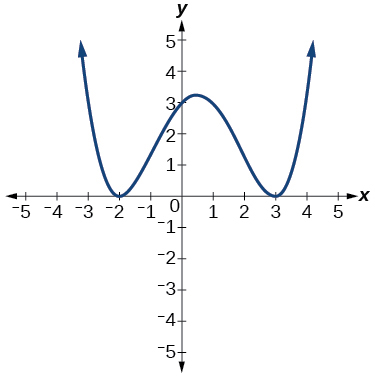–2, 3 each with multiplicity 2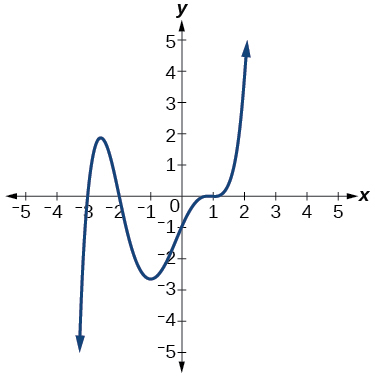For the following exercises, use the given information about the polynomial graph to write the equation.

Degree 3. Zeros at $\text{\hspace{0.17em}}x=–2,$ $\text{\hspace{0.17em}}x=1,\text{\hspace{0.17em}}$ and $\text{\hspace{0.17em}}x=3.\text{\hspace{0.17em}}$ y -intercept at $\text{\hspace{0.17em}}\left(0,–4\right).$

$f\left(x\right)=-\frac{2}{3}\left(x+2\right)\left(x-1\right)\left(x-3\right)$

Degree 3. Zeros at $\text{\hspace{0.17em}}x=\text{–5,}$ $\text{\hspace{0.17em}}x=–2,$ and $\text{\hspace{0.17em}}x=1.\text{\hspace{0.17em}}$ y -intercept at $\text{\hspace{0.17em}}\left(0,6\right)$

Degree 5. Roots of multiplicity 2 at $\text{\hspace{0.17em}}x=3\text{\hspace{0.17em}}$ and $\text{\hspace{0.17em}}x=1\text{\hspace{0.17em}}$ , and a root of multiplicity 1 at $\text{\hspace{0.17em}}x=–3.\text{\hspace{0.17em}}$ y -intercept at $\text{\hspace{0.17em}}\left(0,9\right)$

$f\left(x\right)=\frac{1}{3}{\left(x-3\right)}^{2}{\left(x-1\right)}^{2}\left(x+3\right)$

Degree 4. Root of multiplicity 2 at $\text{\hspace{0.17em}}x=4,\text{\hspace{0.17em}}$ and a roots of multiplicity 1 at $\text{\hspace{0.17em}}x=1\text{\hspace{0.17em}}$ and $\text{\hspace{0.17em}}x=–2.\text{\hspace{0.17em}}$ y -intercept at $\text{\hspace{0.17em}}\left(0,\text{–}3\right).$

Degree 5. Double zero at $\text{\hspace{0.17em}}x=1,\text{\hspace{0.17em}}$ and triple zero at $\text{\hspace{0.17em}}x=3.\text{\hspace{0.17em}}$ Passes through the point $\text{\hspace{0.17em}}\left(2,15\right).$

$f\left(x\right)=-15{\left(x-1\right)}^{2}{\left(x-3\right)}^{3}$

Degree 3. Zeros at $\text{\hspace{0.17em}}x=4,$ $\text{\hspace{0.17em}}x=3,$ and $\text{\hspace{0.17em}}x=2.\text{\hspace{0.17em}}$ y -intercept at $\text{\hspace{0.17em}}\left(0,-24\right).$

Degree 3. Zeros at $\text{\hspace{0.17em}}x=-3,$ $\text{\hspace{0.17em}}x=-2\text{\hspace{0.17em}}$ and $\text{\hspace{0.17em}}x=1.\text{\hspace{0.17em}}$ y -intercept at $\text{\hspace{0.17em}}\left(0,12\right).$

$f\left(x\right)=-2\left(x+3\right)\left(x+2\right)\left(x-1\right)$

Degree 5. Roots of multiplicity 2 at $\text{\hspace{0.17em}}x=-3\text{\hspace{0.17em}}$ and $\text{\hspace{0.17em}}x=2\text{\hspace{0.17em}}$ and a root of multiplicity 1 at $\text{\hspace{0.17em}}x=-2.$

y -intercept at

Degree 4. Roots of multiplicity 2 at $\text{\hspace{0.17em}}x=\frac{1}{2}\text{\hspace{0.17em}}$ and roots of multiplicity 1 at $\text{\hspace{0.17em}}x=6\text{\hspace{0.17em}}$ and $\text{\hspace{0.17em}}x=-2.$

y -intercept at $\text{\hspace{0.17em}}\left(0,18\right).$

$f\left(x\right)=-\frac{3}{2}{\left(2x-1\right)}^{2}\left(x-6\right)\left(x+2\right)$

Double zero at $\text{\hspace{0.17em}}x=-3\text{\hspace{0.17em}}$ and triple zero at $\text{\hspace{0.17em}}x=0.\text{\hspace{0.17em}}$ Passes through the point $\text{\hspace{0.17em}}\left(1,32\right).$

## Technology

For the following exercises, use a calculator to approximate local minima and maxima or the global minimum and maximum.

$f\left(x\right)={x}^{3}-x-1$

local max local min

$f\left(x\right)=2{x}^{3}-3x-1$

$f\left(x\right)={x}^{4}+x$

global min

$f\left(x\right)=-{x}^{4}+3x-2$

$f\left(x\right)={x}^{4}-{x}^{3}+1$

global min

## Extensions

For the following exercises, use the graphs to write a polynomial function of least degree.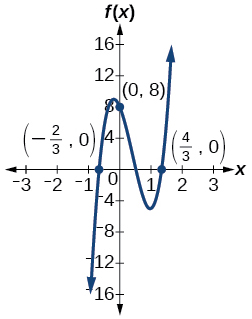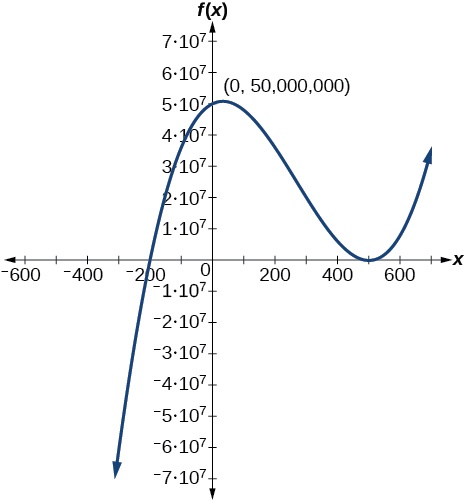$f\left(x\right)={\left(x-500\right)}^{2}\left(x+200\right)$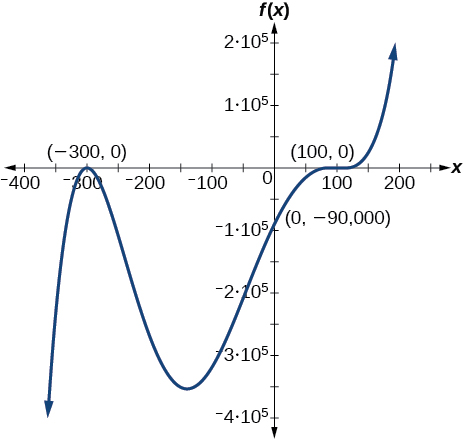## Real-world applications

For the following exercises, write the polynomial function that models the given situation.

A rectangle has a length of 10 units and a width of 8 units. Squares of $\text{\hspace{0.17em}}x\text{\hspace{0.17em}}$ by $\text{\hspace{0.17em}}x\text{\hspace{0.17em}}$ units are cut out of each corner, and then the sides are folded up to create an open box. Express the volume of the box as a polynomial function in terms of $\text{\hspace{0.17em}}x.$

$f\left(x\right)=4{x}^{3}-36{x}^{2}+80x$

Consider the same rectangle of the preceding problem. Squares of $\text{\hspace{0.17em}}2x\text{\hspace{0.17em}}$ by $\text{\hspace{0.17em}}2x\text{\hspace{0.17em}}$ units are cut out of each corner. Express the volume of the box as a polynomial in terms of $\text{\hspace{0.17em}}x.$

A square has sides of 12 units. Squares by units are cut out of each corner, and then the sides are folded up to create an open box. Express the volume of the box as a function in terms of $\text{\hspace{0.17em}}x.$

$f\left(x\right)=4{x}^{3}-36{x}^{2}+60x+100$

A cylinder has a radius of $\text{\hspace{0.17em}}x+2\text{\hspace{0.17em}}$ units and a height of 3 units greater. Express the volume of the cylinder as a polynomial function.

A right circular cone has a radius of $\text{\hspace{0.17em}}3x+6\text{\hspace{0.17em}}$ and a height 3 units less. Express the volume of the cone as a polynomial function. The volume of a cone is $\text{\hspace{0.17em}}V=\frac{1}{3}\pi {r}^{2}h\text{\hspace{0.17em}}$ for radius $\text{\hspace{0.17em}}r\text{\hspace{0.17em}}$ and height $\text{\hspace{0.17em}}h.$

$f\left(x\right)=\pi \left(9{x}^{3}+45{x}^{2}+72x+36\right)$

how fast can i understand functions without much difficulty
what is set?
a colony of bacteria is growing exponentially doubling in size every 100 minutes. how much minutes will it take for the colony of bacteria to triple in size
I got 300 minutes. is it right?
Patience
no. should be about 150 minutes.
Jason
It should be 158.5 minutes.
Mr
ok, thanks
Patience
100•3=300 300=50•2^x 6=2^x x=log_2(6) =2.5849625 so, 300=50•2^2.5849625 and, so, the # of bacteria will double every (100•2.5849625) = 258.49625 minutes
Thomas
what is the importance knowing the graph of circular functions?
can get some help basic precalculus
What do you need help with?
Andrew
how to convert general to standard form with not perfect trinomial
can get some help inverse function
ismail
Rectangle coordinate
how to find for x
it depends on the equation
Robert
yeah, it does. why do we attempt to gain all of them one side or the other?
Melissa
whats a domain
The domain of a function is the set of all input on which the function is defined. For example all real numbers are the Domain of any Polynomial function.
Spiro
Spiro; thanks for putting it out there like that, 😁
Melissa
foci (–7,–17) and (–7,17), the absolute value of the differenceof the distances of any point from the foci is 24.
difference between calculus and pre calculus?
give me an example of a problem so that I can practice answering
x³+y³+z³=42
Robert
dont forget the cube in each variable ;)
Robert
of she solves that, well ... then she has a lot of computational force under her command ....
Walter
what is a function?
I want to learn about the law of exponent
explain this

#### Get Jobilize Job Search Mobile App in your pocket Now!ByByBy David CoreyBy Jesenia WoffordBy Nick SwainBy OpenStaxBy Qqq QqqBy Nicole DuquetteBy Cath YuByBy Madison ChristianBy Megan Earhart